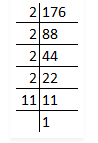# Is 176 a perfect square?

Question:

Is 176 a perfect square? If not, find the smallest number by which it should be multiplied to get a perfect square.

Solution:

First we have to find out the factors by using prime factorisation method.So, prime factors of 176 = 2 × 2 × 2 × 2 × 11

Now, grouping the prime factors = (2 × 2) × (2 × 2) × (11)

= 22 × 22 × 11

Factor 11 has no pair.

∴176 is not a perfect square.

The smallest number it should be multiplied to get a perfect square is 11.

Then,

176 × 11 = 1936

Factors of 1936 = 2 × 2 × 2 × 2 × 11 × 11

= √1936

= √(22 × 22 × 112)

= 2 × 2 × 11

= 44

∴Square root of 1936 is 44.